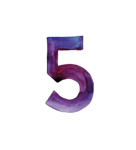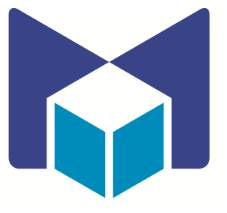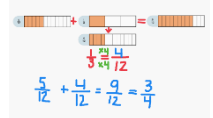Computational Thinker### Overview​

The math learning center is an app and online platform that allows students to use manipulatives virtually. In this activity, students will use virtual manipulatives to add fractions.

Students will:

• Be able to make two fraction addition problems using The Math Learning Center App.

Vocabulary Words:

1. Fraction: A fraction is a part of a whole. A common fraction is made up of a numerator and a denominator. The numerator is shown on top of a line and is the number of parts of the whole. The denominator is shown below the line and is the number of parts by which the whole has been divided.

To prepare for this lesson:

• Teachers will need to have the app or website for virtual manipulatives available to students.

Note: The Math Learning Center has many free apps available for teachers and students. For more information, visit the Math Learning Center

See Accommodations Page and Charts on the 21things4students.net site in the Teacher Resources.

Directions for this activity:

1. Teach students about fractions as parts of a whole. Students should have some prior knowledge of fractions.

2. Take the students to the Math Learning Center

3. Explain to students that when adding fractions, they have to have equivalent parts. For example, if a fraction’s denominator is 12, the fraction it’s added with must also have 12 as the denominator, thus have a common denominator.

4. To make denominators common, you can divide the larger denominator by the smaller and get a multiple.

5. You must multiply the denominator and numerator to make the fractions have common denominators.6. As you show how to create a common denominator to add fractions, have students help you figure out the multiple.

7. Use the annotate feature to label each math sentence.

8. Independent Work: Students take what they have learned about fractions and use the app or website to demonstrate 2 addition problems that they have made up or that are provided by the teacher.

9. Students show teacher their work as they finish.

Note: Early finishers may screenshot their work and save it to their computer or upload to an electronic portfolio like SeeSaw.

Different options for assessing the students:

• Observations
• Check for understanding
• Teachers see what students have done through observation. They can intervene if students are struggling with equivalency.

MITECS: Michigan adopted the "ISTE Standards for Students" called MITECS (Michigan Integrated Technology Competencies for Students) in 2018.

Computational Thinker
5c. Students break problems into component parts, extract key information, and develop descriptive models to understand complex systems or facilitate problem-solving.